# Square Footage Calculator

Find the square footage of an area by selecting a shape and entering the dimensions. Include the price per sq ft to estimate the total cost.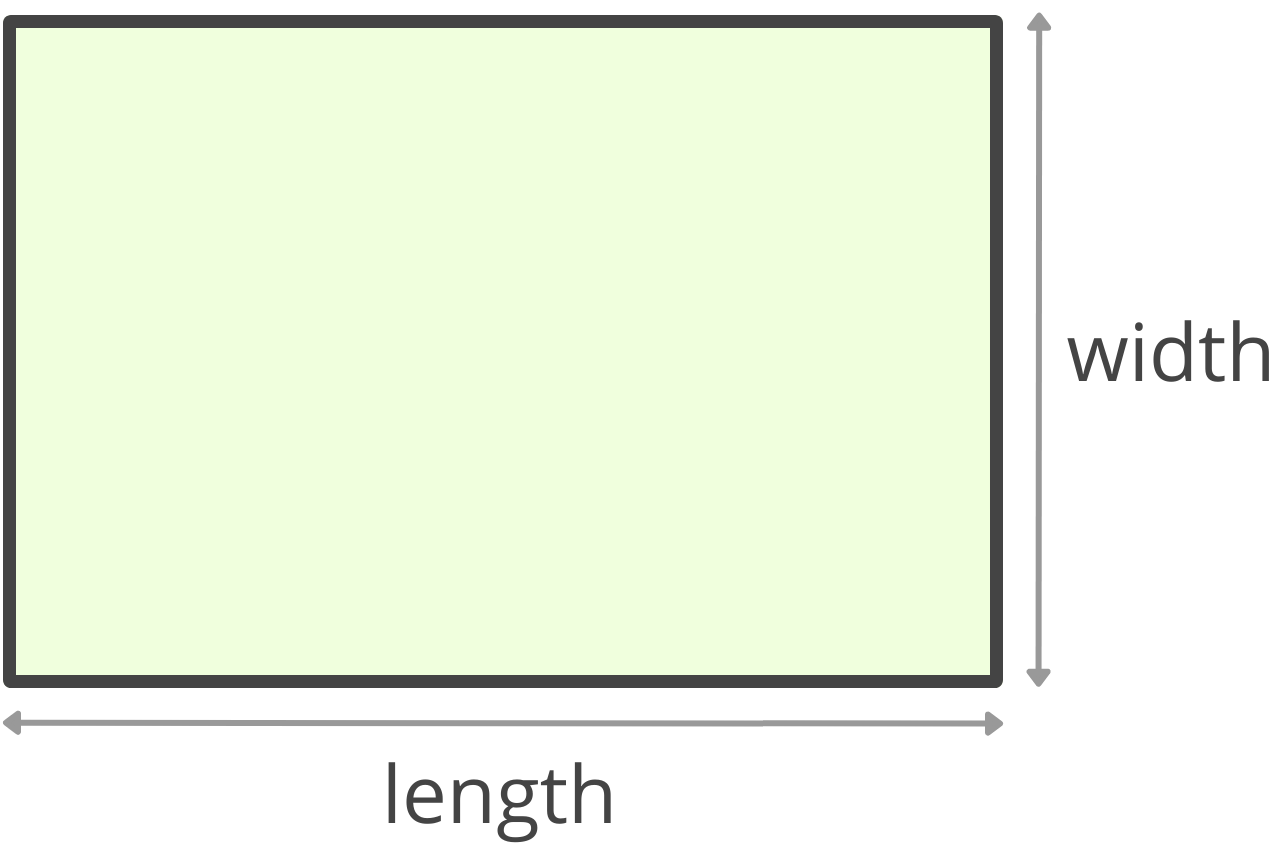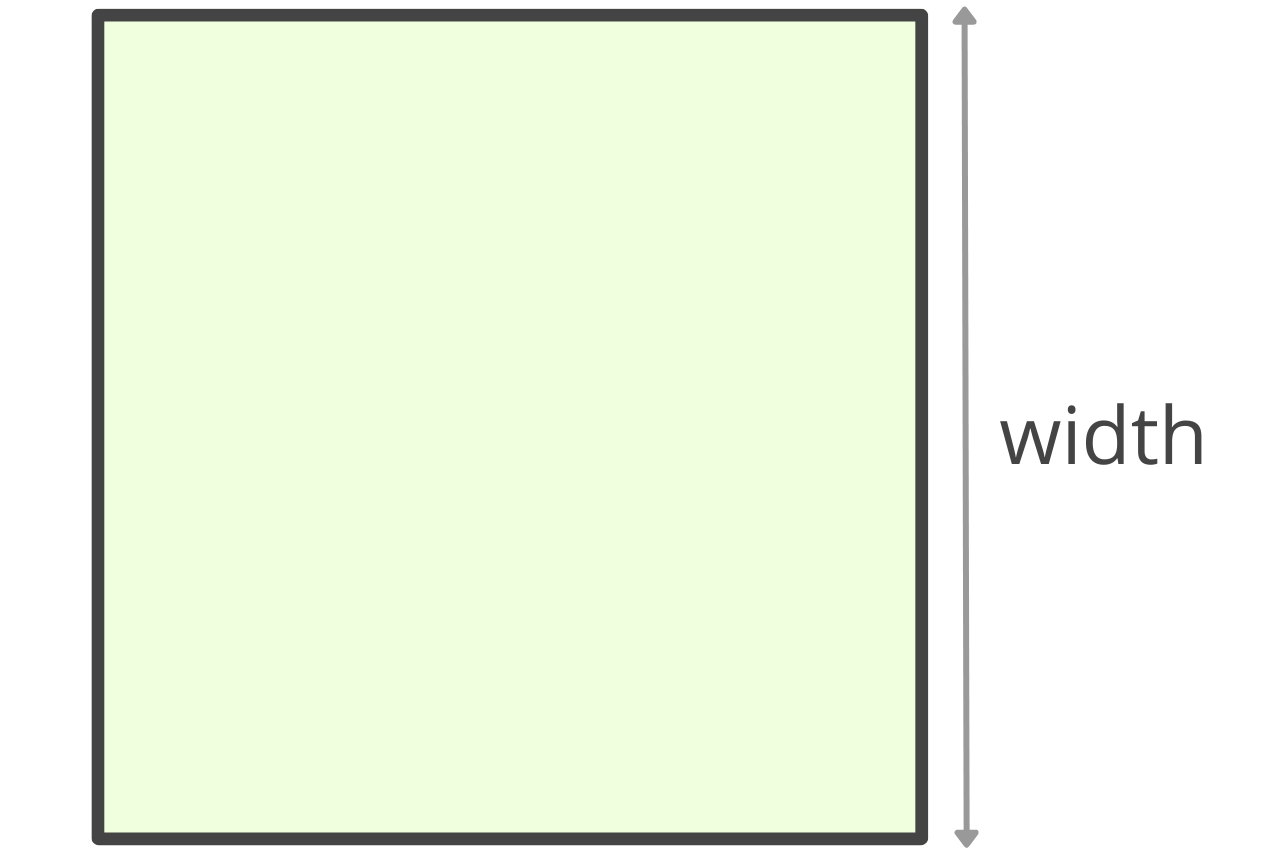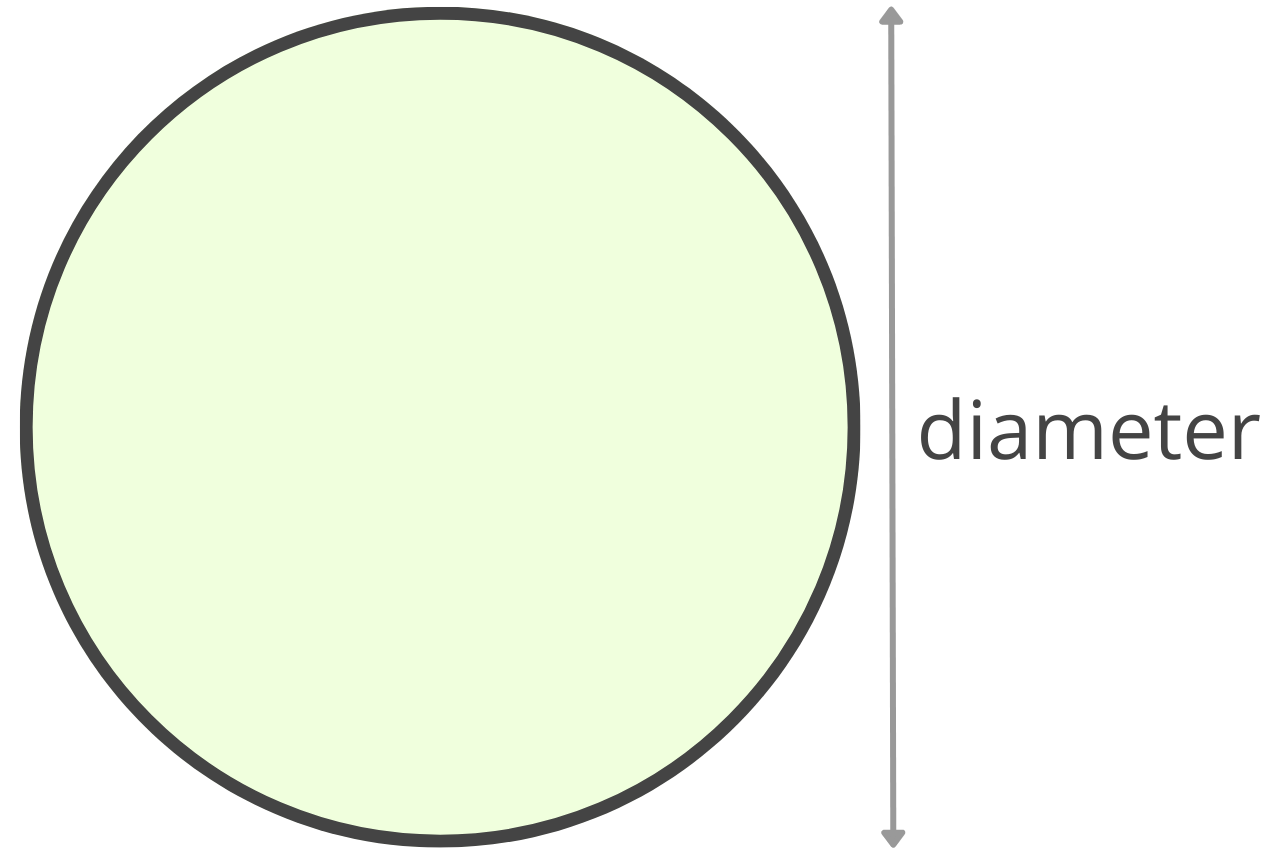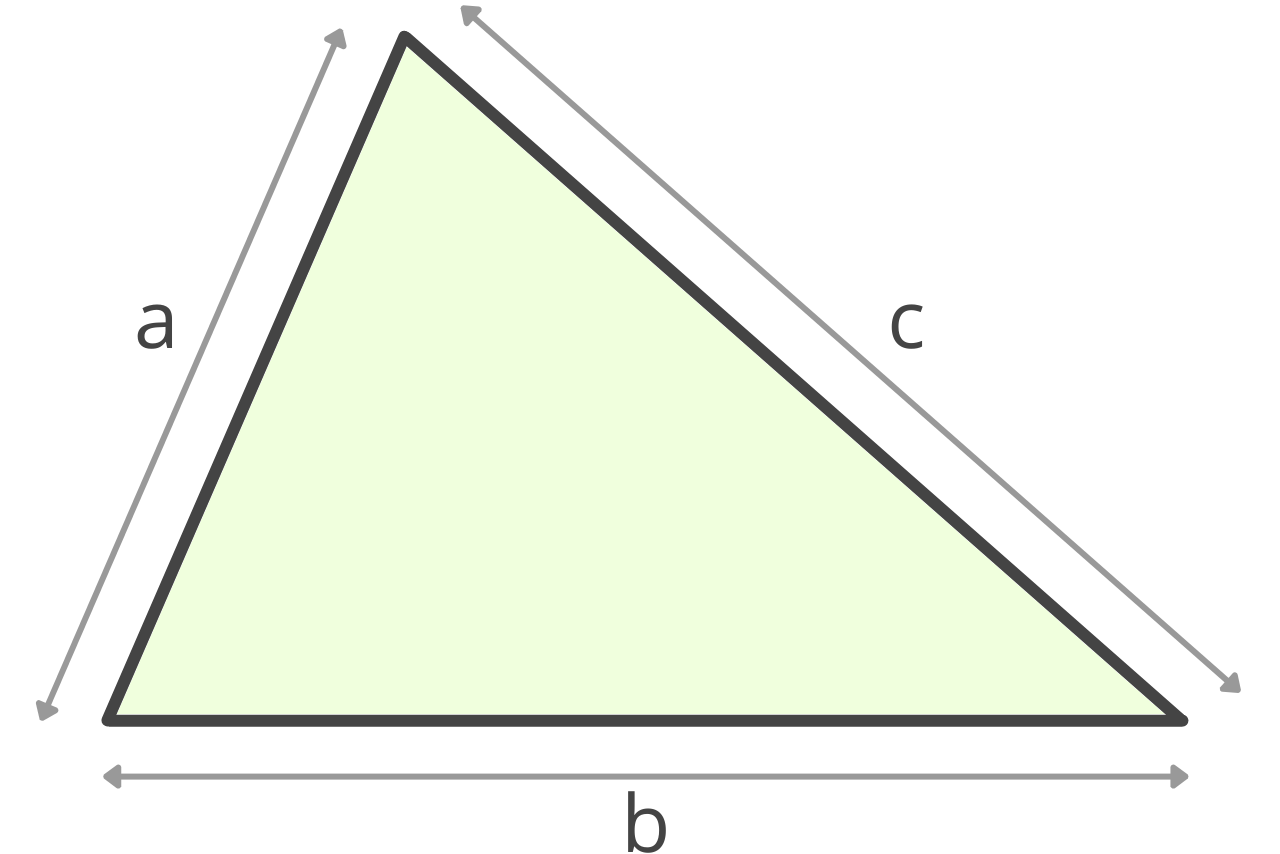Optionally enter the price per square foot
\$

## Area in Square Feet:

### Total Price

\$
Learn how we calculated this below

## How to Calculate Square Footage

Square footage is just an area measured in feet, and it’s often abbreviated sq ft or ft2. One square foot is equal to a square area with a length and width of 1 foot, or 12 inches.

Square footage is commonly used for construction, home remodeling, and renovation projects such as carpeting, hardwood flooring, tiling, drywalling, painting, and gardening material estimates.

You can calculate the square footage of a space using the area formula, which states that the area of a space is equal to its length times its width. This is the formula for a rectangle, but we’ll cover the area formulas for different shapes below.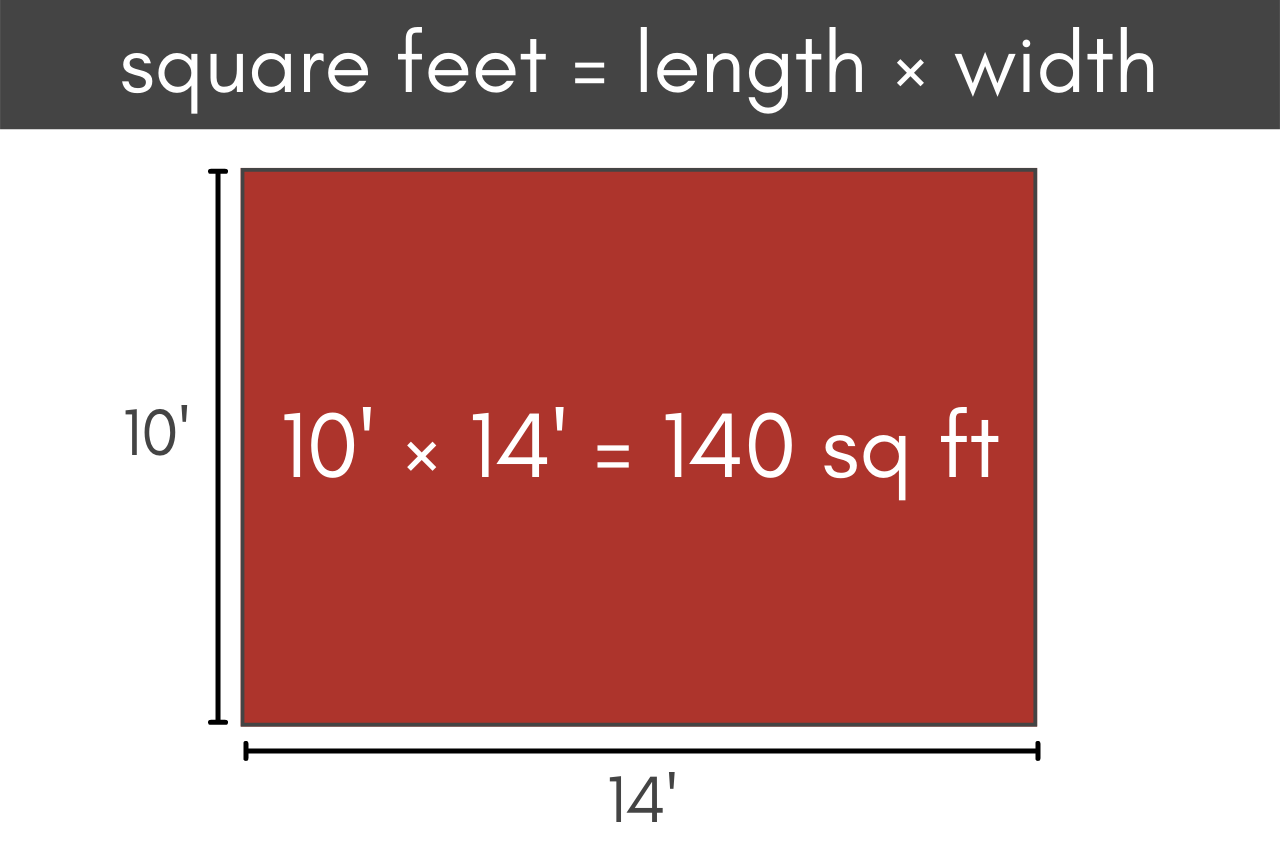Note that to calculate the area in square feet, the length and width must have units of feet. If they are in different units, then before you multiply them together, make sure to convert each measurement into feet.

For example, to calculate the square footage using measurements in inches or another measurement, first convert the length and width measurements to feet by dividing by 12, then multiply them together.

### Steps to Calculate Square Feet

Find the area of a rectangular room or space in square feet by following these easy steps:

• Measure the length and width of the area.
• Convert these measurements to feet if they aren’t already. Convert inches, yards, or meters to feet using a converter if needed.
• Multiply the length in feet times the width in feet (length × width). Use one of the formulas below to calculate square footage for various non-rectangular shapes.
• To estimate the cost of materials to cover the area, multiply the total square footage by the price per square foot.
• If the area is not a simple shape, break it into manageable parts and calculate the area of each part separately and then add them together. For example, to measure a floor of your home, calculate the square feet of each room, then add all area measurements together to derive the total square footage.

For example, let’s find the square feet of a room that is 12′ wide by 16′ long.

area = 12′ × 16′
area = 192 sq ft

### Square Footage Formulas

Use the formulas below to find the area in square feet for various shapes. Convert all measurements to feet before using the formulas.

#### Rectangle

sq ft = l × w

l = length
w = width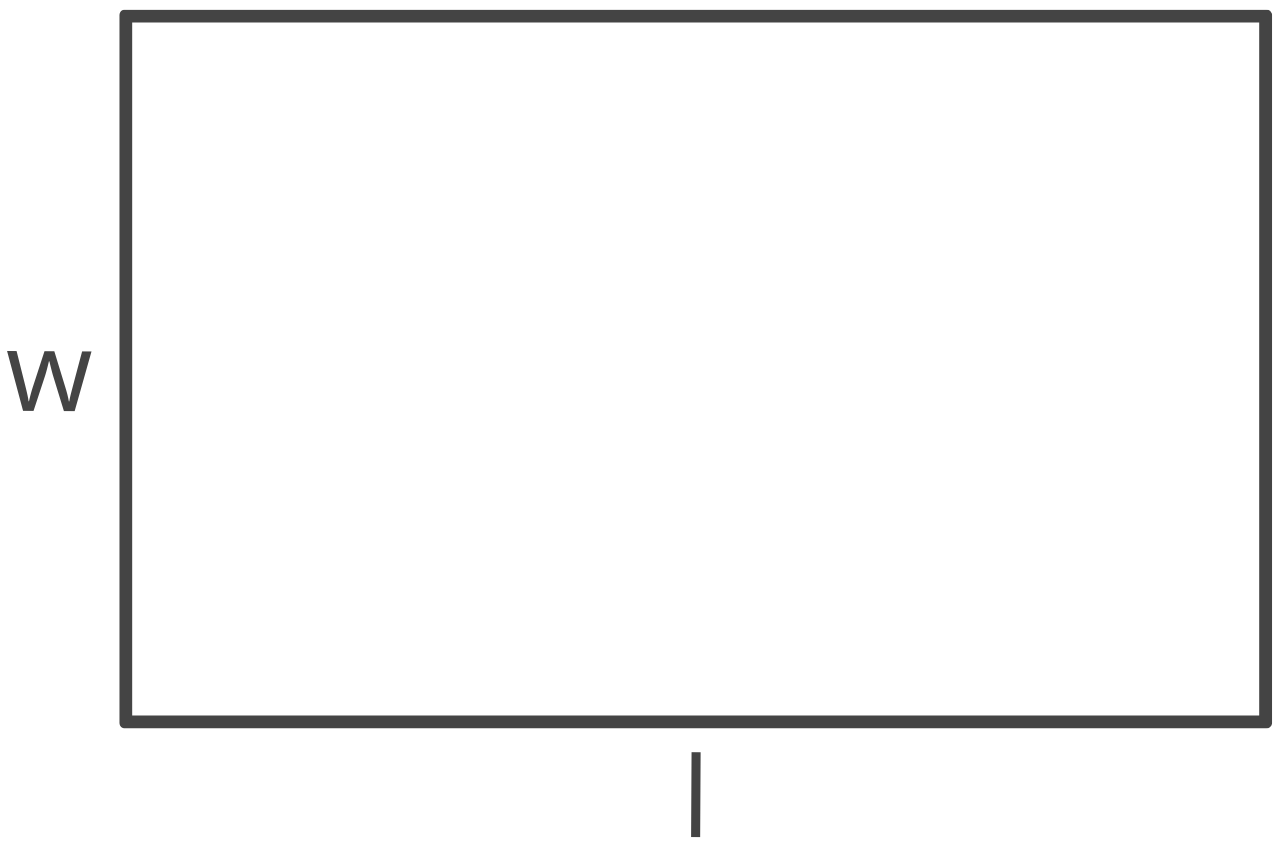#### Rectangular Border

sq ft = (l × w) – (a × b)

l = outer length
w = outer width
a = inner length
b = inner width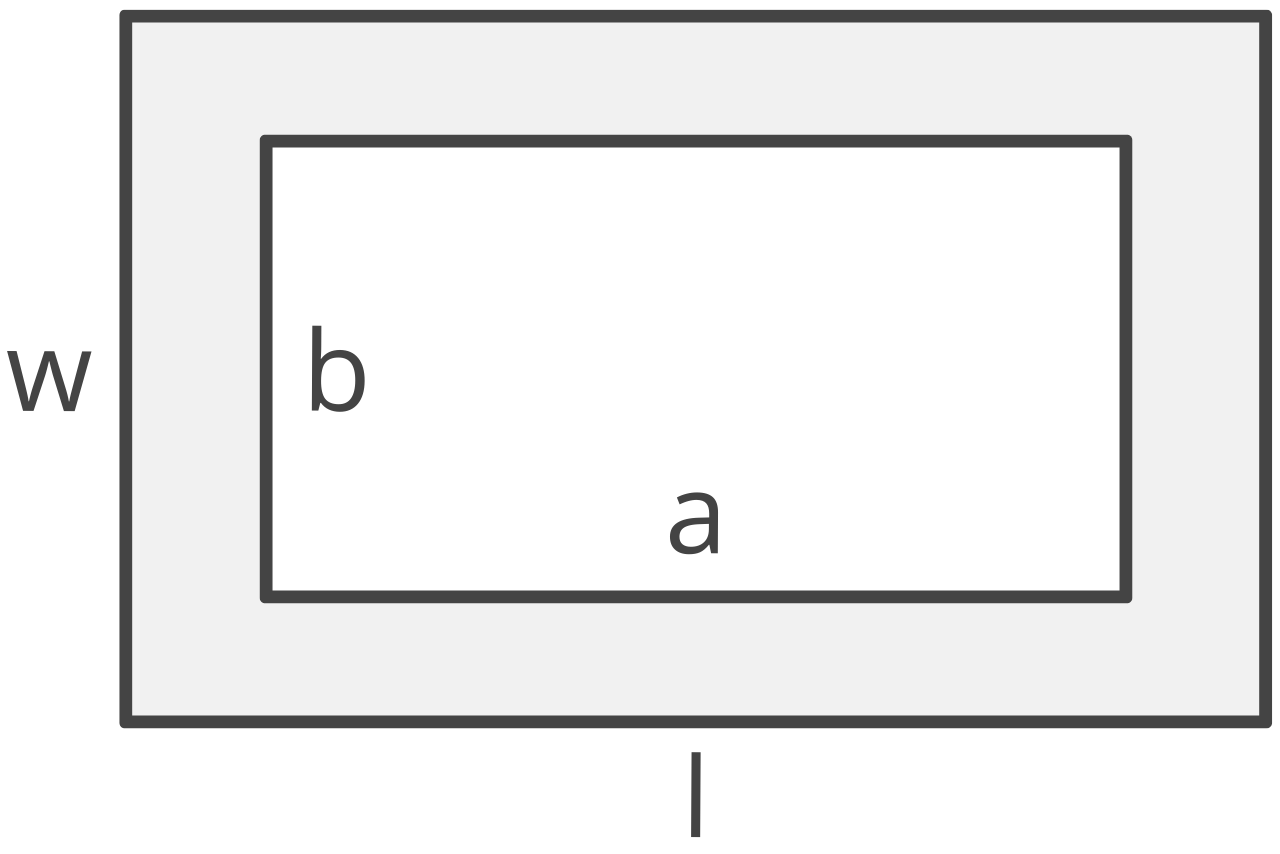#### Circle

sq ft = πr2

π = 3.14159265359

If you know the diameter of the circle, you can find the radius by dividing the diameter in half.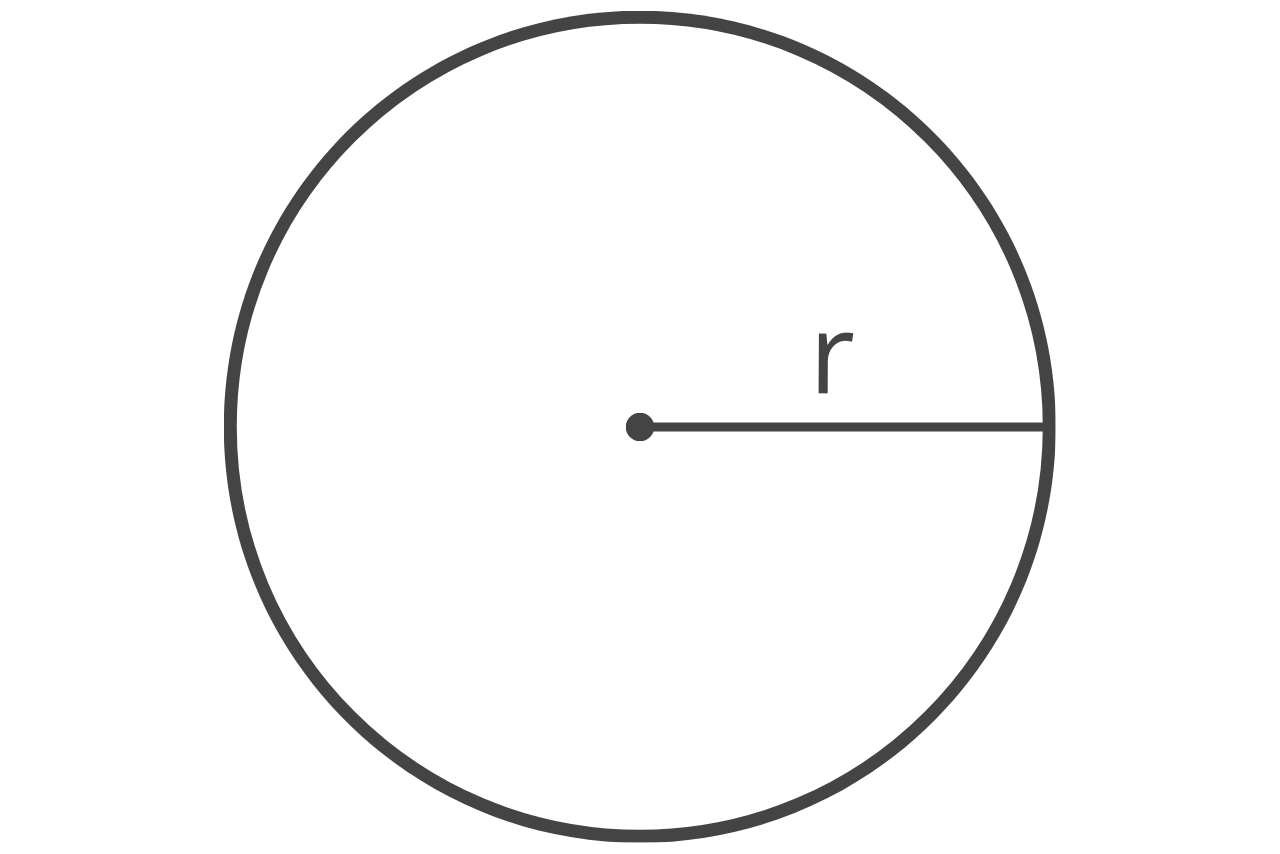#### Triangle

sq ft = bh/2

b = base
h = height

If you don’t know the height of the triangle, you can find it using the length of the three sides.

s = 1/2(a + b + c)
sq ft = s(s – a)(s – b)(s – c)

a = edge a
b = edge b
c = edge c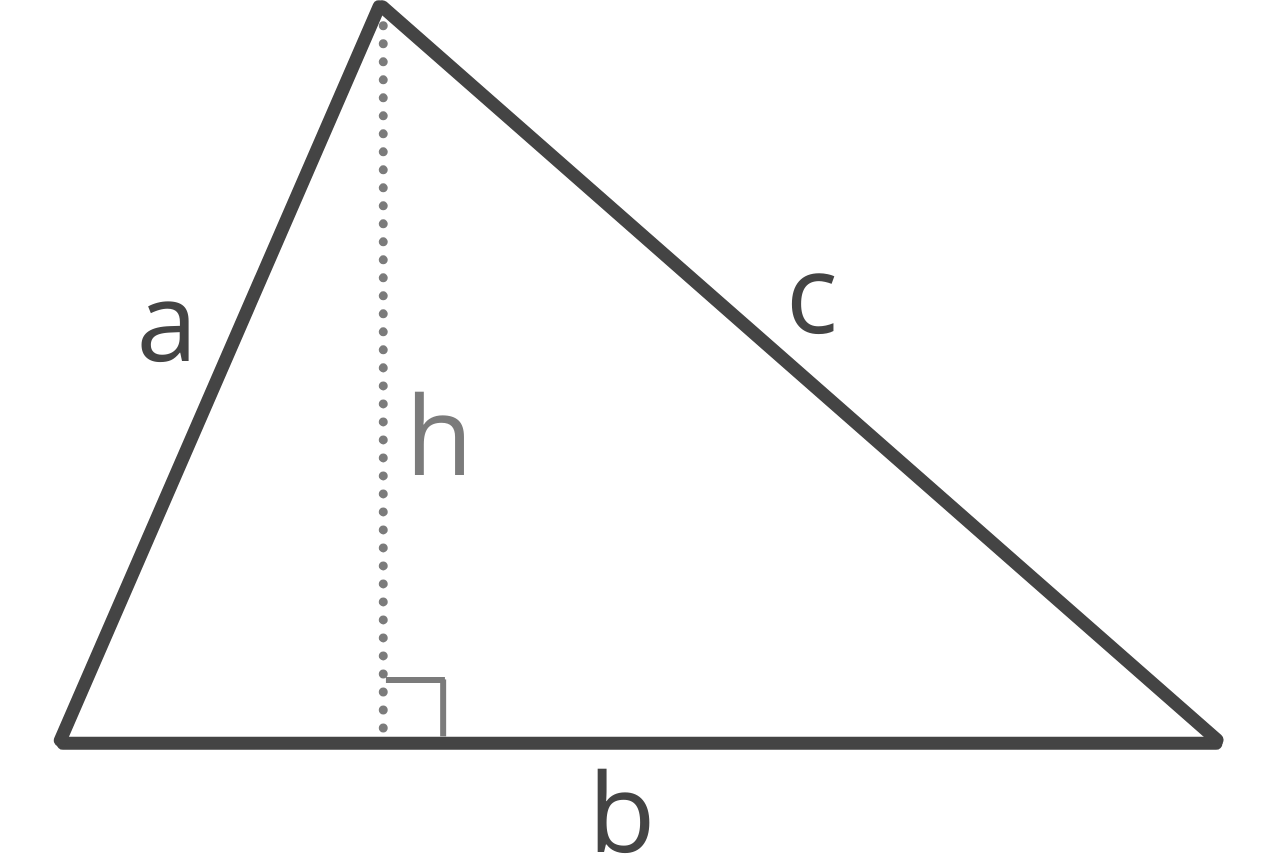Our area calculator has even more formulas for different shapes.

## How to Find the Square Footage of a Room

You can use the formulas above to find the square footage of any room in your home. Measure the dimensions of the room using a tape measure, then apply them to the applicable formula.

For instance, if the room is rectangular, measure the length and width in feet. Then, multiply them together to find the area in square feet.

For oddly shaped rooms, split the room into smaller, easily measurable sections, and calculate the area of each section separately. Add the area of each section together to find the total square footage of the room.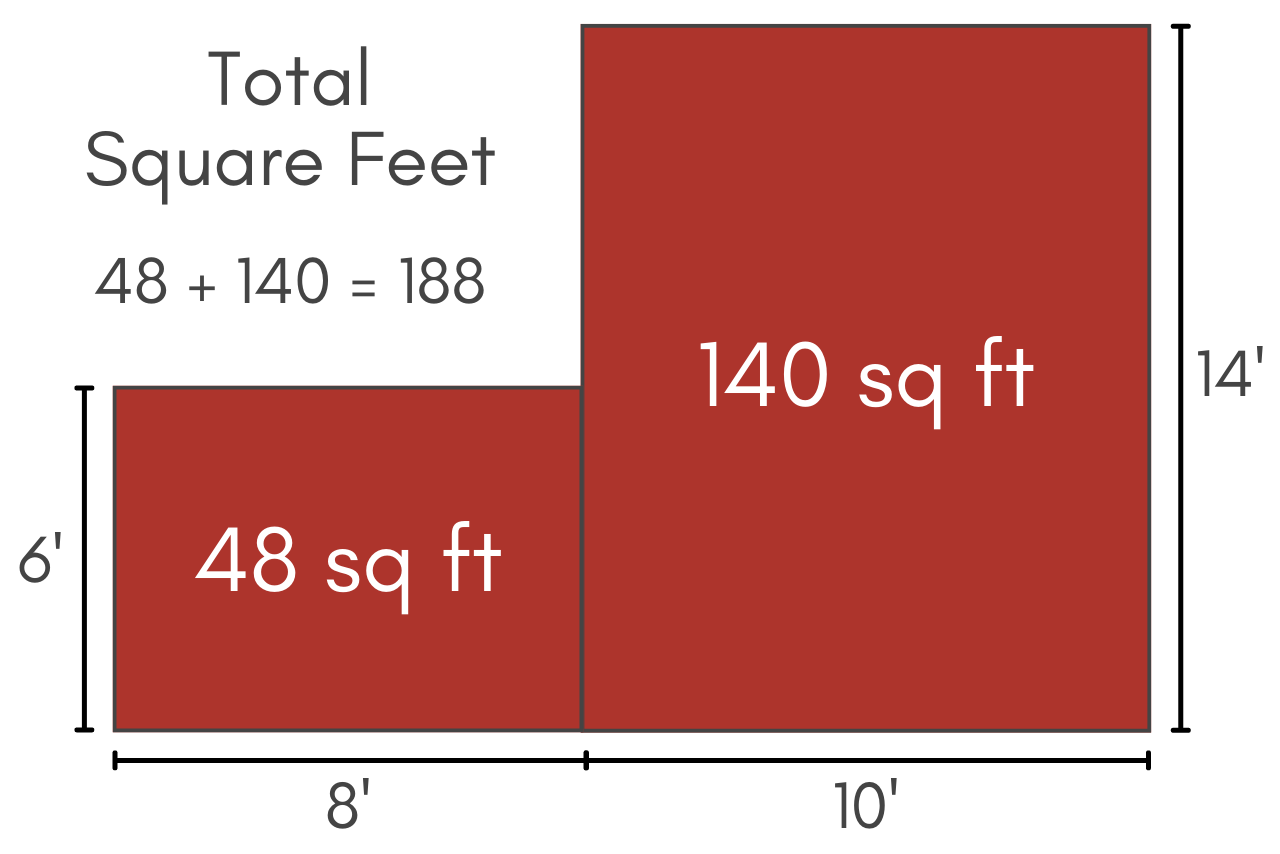## How to Calculate the Square Feet of a Home or Residence

As noted above, measuring the square footage of a house is important in real estate and for home improvements. Unlike measurements for a flooring project, when you’re measuring the area of a home or residence for real estate purposes, some special considerations apply to determine the inhabitable square footage.

Since the livable size of a home determines the market value, appraisal price, and property taxes, getting an accurate measurement is important.

Only livable rooms with finished walls, floor, and ceiling count toward the finished area of the home. For a room to be considered livable, the space should be finished and should have heating or air conditioning as applicable.

Indoor spaces like bedrooms and closets count toward the square footage of a home, while outdoor spaces such as a balcony or garage typically do not. In fact, the American National Standards Institute has defined rules for what counts as a finished area and which rooms contribute to the gross living area (GLA) of a home.

To calculate the total square footage of a home, measure each room in feet using a tape measure. Then, for each room, calculate the area using the appropriate formula depending on its shape.

Finally, add the area measurements together to find the total square footage. The calculator above can help find the square footage of one or more rooms using this method.

We also have great resources on how to measure rooms and complex spaces.

### How to Calculate Price per Sq Ft

To calculate the price per square foot of your home, divide the total price of the home by the total number of square feet.

price per ft2 = total price ÷ total ft2

For example, to find the price per ft2 of a home that costs \$200,000 and is 2,000 ft2, use this formula.

price per ft2 = \$200,000 ÷ 2,000 ft2
price per ft2 = \$100

If you’d prefer not to do the math, you can also use our unit price calculator to calculate the price per square foot.

## How to Convert to Square Feet

You can also calculate square feet by converting a known area that’s in different units of measure. You can convert square inches, square yards, or square meters to square feet using one of the formulas below.

Square Inches:
sq ft = sq in ÷ 144

Square Yards:
sq ft = sq yds × 9

Square Meters:
sq ft = sq m × 10.764

You can use an area converter to convert any area measurement to sq ft.

### Are square feet and feet the same?

No, feet are a one-dimensional unit of length, while square feet are a two-dimensional unit of area.

### What does 1 square foot look like?

One square foot is equal to the space taken up by a 1 ft by 1 ft square. You can visualize this by making a square out of 4 standard 12-inch rulers.

### When do you use square feet?

Square feet are used any time you want to represent the area of a space. It is most commonly used in measuring the living space of homes, but is also commonly used in landscaping and commercial real estate.

### How many square feet is a 12x12 room?

A 12 ft by 12 ft room is equal to 144 sq ft:
12 ft × 12 ft = 144 sq ft

## References

1. American National Standards Institute, Square Footage — Method for Calculating, ANSI, Z765-2021, approved March 2021,
2. Fannie Mae, Standardized Property Measuring Guidelines, December 2021, https://singlefamily.fanniemae.com/media/30266/display